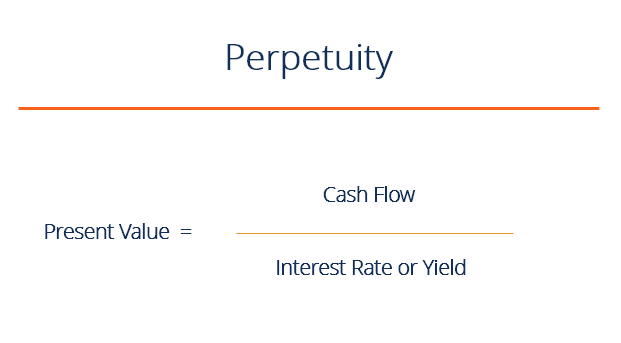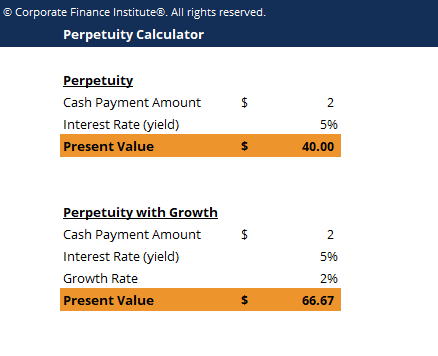# Perpetuity

An indefinite cash flow payment

## What is a perpetuity?

A perpetuity in the financial system is a situation where a stream of cash flow payments continues indefinitely or is an annuity which has no end. In valuation analysis, perpetuities are used to find the present value of a company’s future projected cash flow stream and the company’s terminal value. Essentially, a perpetuity is a series of cash flows that keep paying out forever.### Finite present value of perpetuity

Although the total value of a perpetuity is infinite, it has a limited present value. The present value of an infinite stream of cash flow is calculated by adding up the discounted values of each annuity and the decrease of the discounted annuity value in each period until it reaches close to zero.

The finite present value of a perpetuity is used by an analyst to determine the exact value of the company if it continues to perform at the same rate.

### Real-life examples

Although a perpetuity is somewhat theoretical (can anything really last forever?), classic examples include businesses, real estate, and certain types of bonds.

One of the examples of a perpetuity is the UK’s government bond, known as a Consol.  Bondholders will receive annual fixed coupons (interest payment) as long as they hold the amount and the government does not discontinue the Consol.

The second example is in the real-estate sector when an owner purchases a property and then rents it out. The owner is entitled to an infinite stream of cash flow from the renter as long as the property continues to exist (assuming the renter will rent)

Another real-life example is preferred stock; the perpetuity calculation assumes the company will continue to exist indefinitely in the market and keep paying dividends.

### Present value of perpetuity formula

Here is the formula:

PV = C / R

Where:

PV = Present value

C = Amount of continuous cash payment

r = Interest rate or yield

### Example – Calculate the PV of a Constant Perpetuity

Company “Rich” pays \$2 dividends annually and estimates they will pay it indefinitely. How much are investors willing to pay for the dividend with a required rate of return of 5%?

PV = 2/5% = \$40

An investor will consider investing in the company if it’s worth \$40 or less.Enter your name and email in the form below and download the free template now!

### Perpetuity with growth formula

Formula:

PV = C / (r – g)

Where:

PV = Present value

C = Amount of continuous cash payment

r = Interest rate or yield

g = Growth Rate

### Example calculation

Taking the above example, imagine if the \$2 dividend is expected to grow annually by 2%.

PV = \$2 / (5 – 2%) = \$66.67

### Importance of a growth rate

The growth model is important for some terminal value calculations in the discounted cash flow model. The last, or terminal year, in the DCF model, will be assumed to grow at a constant rate forever. This, in essence, means that the terminal year cash flow is a continues stream of cash flow.

Thank you for reading this guide to perpetuities. CFI is the official provider of the Financial Modeling and Valuation Analyst (FMVA) designation, designed to transform anyone into a world-class financial analyst. To help on your journey, these additional resources will be helpful:

• Valuation Methods
• Cost of Preferred Stock
• Market Risk Premium
• Debt Schedule

### Financial Analyst Training

Get world-class financial training with CFI’s online certified financial analyst training program!

Gain the confidence you need to move up the ladder in a high powered corporate finance career path.

Learn financial modeling and valuation in Excel the easy way, with step-by-step training.